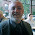### Cursing and re-cursing!

So last night, tired of writing a new Python function whenever I wanted to write a new recurrence test question, I wrote a recurrence harness. It turns a handful of basic lines of code into two, but more importantly, because I can re-use the harness it is worth spending the time to write proper error checking and to memoize it. Here is the code.

Below here are some examples. Note that, because this is memoized, we get the 4000th Fibonacci number essentially instantly, while without memoization, the runtime increases faster than the Fibonacci sequence itself, so, even if we could do one recursive call per nanosecond, we would be looking at roughly 1.2 * 10819 years for the recursive version to finish... and that's a long time for students to wait for their final to be graded.

The 4000th Fibonacci number:
fibb = {0: 0, 1: 1}
def fibf(n, bases):
return recur(n - 1, bases, fibf) + recur(n - 2, bases, fibf)

In : recur(4000, fibb, fibf, True)
Out: 39909473435004422792081248094960912600792570982820257852628876326523051818641373433549136769424132442293969306537520118273879628025443235370362250955435654171592897966790864814458223141914272590897468472180370639695334449662650312874735560926298246249404168309064214351044459077749425236777660809226095151852052781352975449482565838369809183771787439660825140502824343131911711296392457138867486593923544177893735428602238212249156564631452507658603400012003685322984838488962351492632577755354452904049241294565662519417235020049873873878602731379207893212335423484873469083054556329894167262818692599815209582517277965059068235543139459375028276851221435815957374273143824422909416395375178739268544368126894240979135322176080374780998010657710775625856041594078495411724236560242597759185543824798332467919613598667003025993715274875

# the value of an annuity with equal contributions each year
#  and interest compounded annually:
INT_RATE = .05
INITIAL = 1000     # redefine INITIAL to get different yearly amount
compb = {0: INITIAL}
def compf(n, bases):
return compb + recur(n - 1, bases, compf) * (1 + INT_RATE)

In : recur(10, compb, compf, True)
Out: 14206.787162326274

# an arbitrary recurrence:
arbb = {0: 1.01, 1: .98, 2: .88, 3: 1.1}
def arbf(n):
return recur(n - 1, arbb, arbf) * recur(n - 4, arbb, arbf)

In : recur(4, arbb, arbf, True)
Out: 1.1110000000000002

In : recur(5, arbb, arbf, True)
Out: 1.08878

In : recur(6, arbb, arbf, True)
Out: 0.9581264

In : recur(7, arbb, arbf, True)
Out: 1.0539390400000002

In : recur(8, arbb, arbf, True)
Out: 1.1709262734400003

1.I think you get an error if n = MAX_RECURS, so your test in the elif should be >= I think.

1.The thing is, I set the max Python depth to n, and then MAX_RECURS to (if I recall) n / 2. That's because it ain't precise: it all depends on what the particular recurrence the user feeds in does. It's just a guess as to how far one might recurse... if instead the Python stack blows... well, you find out you went to far that way instead!